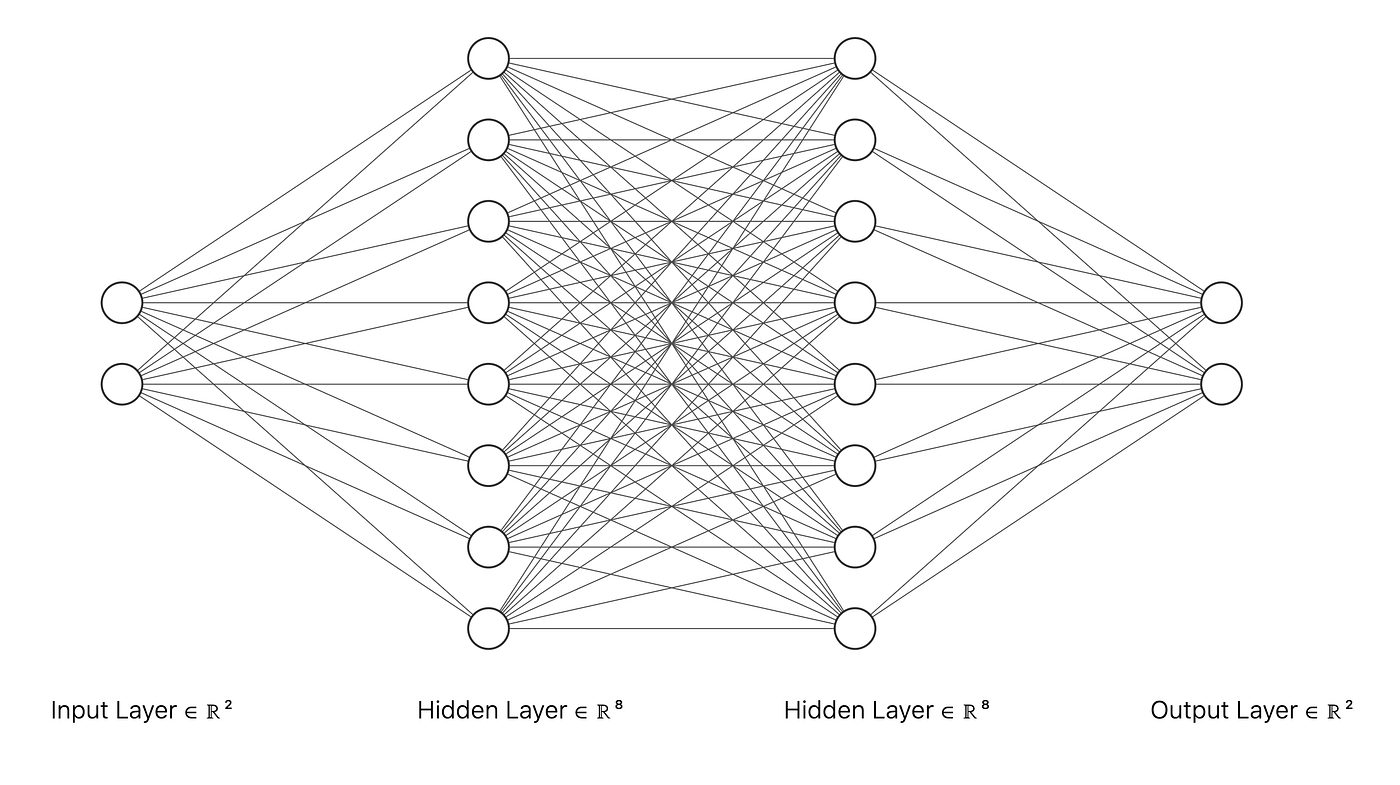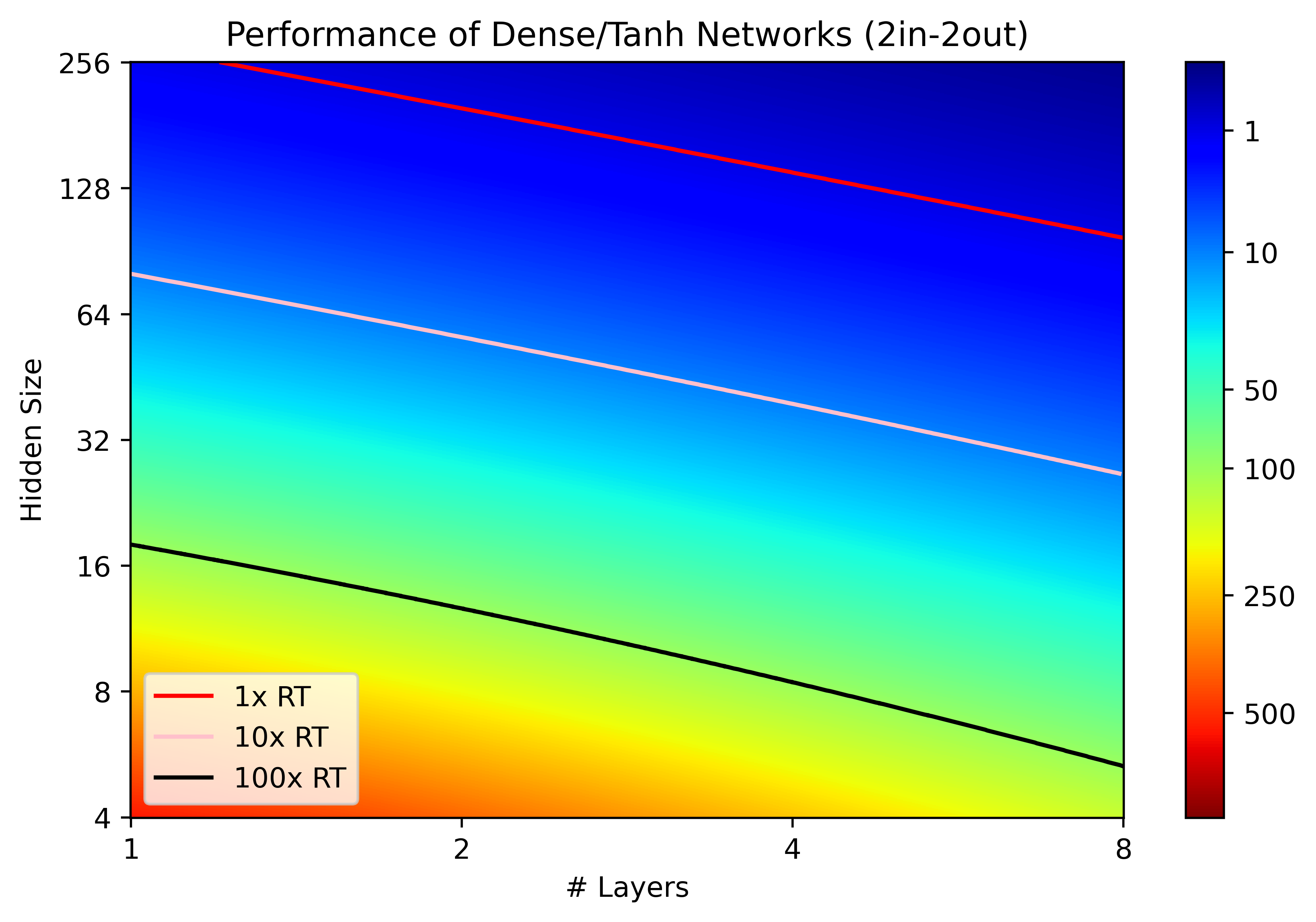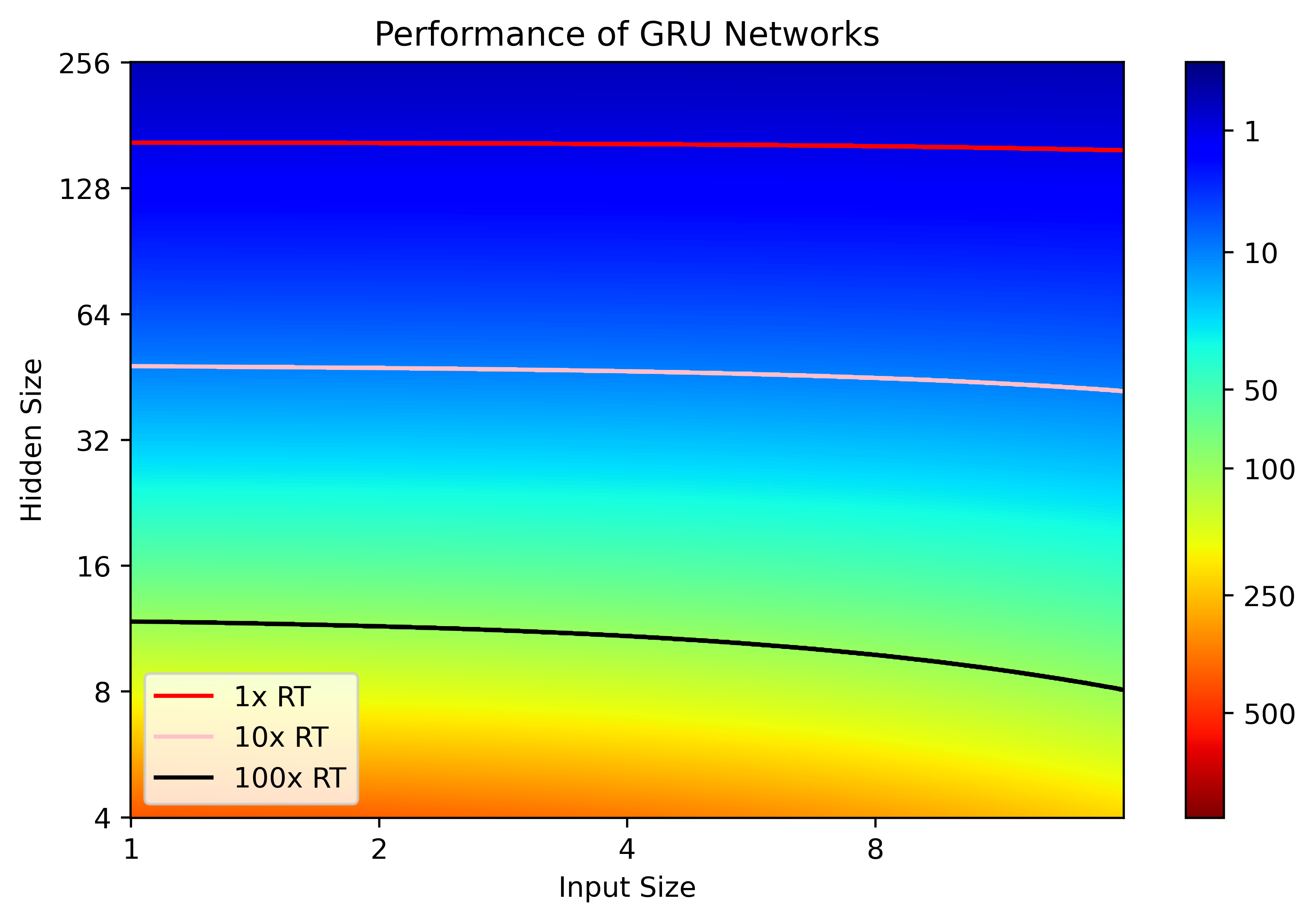# Quantifying Performance

The most fundamental way to quantify performance for any system that is intended to run in real-time is if it is fast enough to meet the real-time “deadline”. For example, in a typical audio system, a neural network might be asked to process or produce samples at a rate of 48000 samples per second. With that in mind, the real-time deadline for a neural network that processes individual samples would be 0.02 milliseconds. If a neural network requires more time to process a single sample, then it is not fast enough to run in real-time.

## What’s A Good Score?

In real-time audio systems, the performance constraints are highly dependent on the context in which the system is deployed. In the context of audio plugins, most users expect to be able to run many plugins simultaneously, meaning that any neural network inferencing must happen fast enough to allow for other processes to happen without going past the real-time deadline. For plugins, I usually try to aim for a real-time score greater than 10x, at a 48 kHz sample rate, meaning that the user could run 10 instances of the plugin on a single thread without going past the real-time deadline.

# Predicting Performance

Now let’s think about how we could predict the performance of a neural network. In signal processing literature, one common way to compare the performance of various algorithms is to count the operations used by each algorithm and compare. However, there are a few difficulties with this form of comparison. For instance, it is generally accepted that some operations (like multiplication/division) are more expensive than other operations (like addition/subtraction), but it is often unclear exactly how much more expensive. Is a multiply operation 10x more expensive than addition? 20x? 100x? Further, this approach fails to consider that when the algorithm is implemented in code, some of the multiplies or additions may be combined into “vectorized” operations using SIMD instructions.

• Count the operations used by the neural network as a function of the network hyper-parameters.
• Measure the network performance for a variety of hyper-parameter choices.
• Use a regression to estimate how long each operation will take.

## Example: Dense NetworkVisualization of a Dense network with 2 inputs, 2 outputs, 2 hidden layers, and a hidden size of 8.
• SIMD Adds: ((L + 1) * W + O) / V
• SIMD Activations: (L + 1) * W / V
• SIMD Adds: 1.47277176e-25 seconds
• SIMD ReLU Activations: 7.23480113e-26Real-Time Factor for Dense/ReLU and Dense/Tanh networks of a given size. Networks that fall above the red line are too slow to run in real-time at 48 kHz.

## Example: Recurrent Networks

As another example, let’s look at recurrent neural networks, which have been popular for modelling stateful nonlinear systems in audio signal processing, typically using one or more input sample and a single output sample at each time step.Real-Time Factor for LSTM and GRU networks of a given size.

# Why Is This Useful?

Now that we’ve seen one potential way for predicting the performance of a real-time neural network, it’s worth asking how this information can be useful for us in training and implementing these types of networks. I think the main significance of this analysis is in selecting network architectures for training and implementation.

# Conclusion

I hope sharing this information and the process for analyzing and predicting real-time neural network performance is useful. Feel free to contact me if you have any questions about the code or data related to the examples shown here!

--

--